## Quiz 5 : Background to SupplyLooking for Economics Homework Help?# Quiz 5 : Background to Supply

Degrees of Price Elasticity of Demand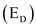a.A firm's demand curve for its product is elastic , at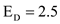. With elastic demand curve, if the firm raises the price of its product, then its total revenue will decrease. b.A firm's demand curve for its product is unitary elastic , at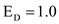. With unitary elastic demand curve, if the firm raises the price of its product, then its total revenue will not change. c.A firm's demand curve for its product is perfectly elastic , at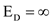. With perfectly elastic demand curve, if the firm raises the price of its product, then its total revenue will be zero. d.A firm's demand curve for its product is inelastic , at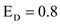. With inelastic demand curve, if the firm raises the price of its product, then its total revenue will increase.

Relationship between Price Elasticity of Demand and Total Revenue Total revenue is negatively related to the price elasticity of demand when price elasticity of demand is greater than 1;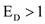. If demand is elastic, increase in price will raise the total revenue and vice-versa.Total revenue is positively related to the price elasticity of demand when price elasticity of demand is less than 1;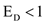. If demand is inelastic, increase in price will raise the total revenue and vice-versa.There is no effect on total revenue when price elasticity of demand is equal to 1;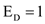.

Linear Demand Curve: Price Elasticity Price elasticity of demand is defined as percentage change in quantity demanded divided by the percentage change in corresponding price. Quantity demanded for a good is more when its price is low and vice-versa.When price of a good is low, then its quantity demanded is quite large. Any further fall in price will cause less proportionate increase in the quantity demanded. Thus, proportionate fall in price is more than the proportionate increase in quantity demand, that is, the price elasticity is low. Similarly, when the price of a good is quite high, then its quantity demanded is low. Any further increase in price will cause more proportionate fall in the quantity demanded. Thus, proportionate increase in price is less than the proportionate fall in quantity demand, that is, the price elasticity is large.Thus, many price elasticities can be associated with a single demand curve.